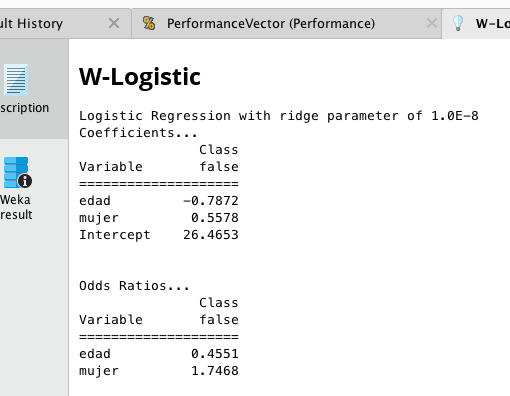# New Logistic Regression Operator in Version 7.3

Member Posts: 270Unicorn
edited November 2018 in Help

I'm glad finally there is an operator in Rapidminer to estimate standard logistic regression. My question has to do with the class defined as "success". Let's say I have a binomial label  Y with two possible outcome "A" or "B".  Which equation is being estimated by RM:

P( Y = "A" | regressors ) = Exp( beta*X) / (1 + Exp(beta*X) )

or

P( Y = "B" | regressors ) = Exp( beta*X) / (1 + Exp(beta*X) ).

Before this operator became available I used the Weka operator W-Logistic. The output of this operator indicated the Class used as "success" (for instance in the output below the modeled class is "false".Tagged: